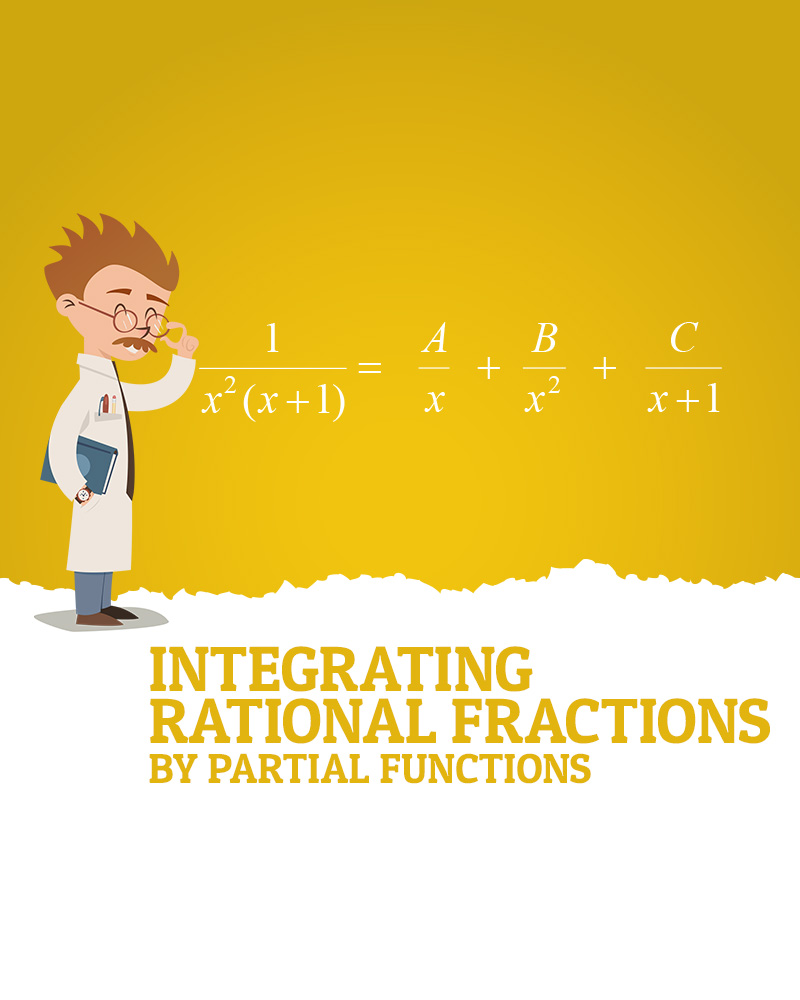# Integrating Rational Fractions by Partial Fractions

If you take a look at the integrand of the question, it seems a relatively complicated fraction.  If we can split it into simpler fractions, then we may be able to integrate them easily.

Making use of partial fractions to get the simpler fractions.

First, we need to have factored form of the denominator to make partial fractions as we need to split the fraction by separating the factors.

Taking the common factor x^2 out to get x^2 (x+1) for the denominator.  We should consider x^2 as x is repeated.